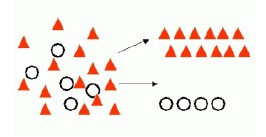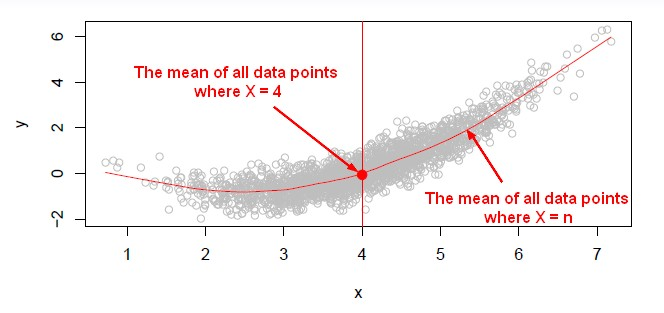# Machine Learning - Rote Classifier

The “rote” classifier classifies data items based on exact matches to the training set. Otherwise, it search in the training set for one that’s “most like” it. The key concept here is the description of what means “most like” (for instance: randomly)

It's the simplest form of learning.

It's a algorithm based on distance calculation from the training data set.

The training instances are the data structures and represent the “knowledge”. The representation of knowledge is then just the set of instances.

There is then no model. It's a kind of lazy algorithm. It's a lazy learning algorithm as it will do nothing until you have to make predictions.

## Algorithm

It just remembers the training instance and then to classify a new instance it search the training set for one that is “most like” a new instance.

### Randomly

“Most like” algorithm as a pure guess:

``````If the record exactly match a previous record then
assign the same class as the previous record
else
just guess
```
```

### k nearest neighbour

Discover MoreData Mining - (Classifier|Classification Function)

A classifier is a Supervised function (machine learning tool) where the learned (target) attribute is categorical (“nominal”) in order to classify. It is used after the learning process to classify...Machine Learning - K-Nearest Neighbors (KNN) algorithm - Instance based learning

“Nearest‐neighbor” learning is also known as “Instance‐based” learning. K-Nearest Neighbors, or KNN, is a family of simple: classification and regression algorithms based on Similarity...